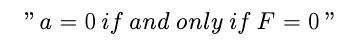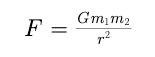# Physics : Newton's Laws of Rectilinear Motion : First Law : (Relation of force and Motion)

Newton's Laws of Rectilinear Motion : First Law : (Relation of force and Motion)

A law govering the relationship between forces and motion was completly described by Sir Issac Newton on the basis of inertia law of Galileo which asserted that if there are no external actions on a body it neither moves with a constant velocity or is at rest.Newton formulated the law as fallow :

" A body will remain in its state of rest of uniform rectilinear motion (having zero acceleration) unless it is compelled to change its state by application external force "'

OR

If the (Vector) sum of all thee forces acting on a given particle is zero then and only then the particle remains unaccelarated (i.e remains at rest or moves with constant velocity)

If the sum of all forces on a given particle is F and its acceleration is a, the above statement many also be written asThus, if the sum of the forces acting on a particle is known to be zero, we can be sure that the particle is unaccelerated , or if we know that a particle is unaccelerated, we can be sure that the sum of forces acting on the particle is zero.

If no external forces acts on a system, the state of system does not change.Here,

State  -----> Centre of Mass

If system consist of more the one particle then newton's law is collectivly applied to centre of Mass.

Post By : Rahul Kumar 23 May, 2020 693 views Physics